# Simultaneous Equations

Welcome to class!

In today’s class, we will be talking about simultaneous equations. Enjoy the class!

### Simultaneous Equations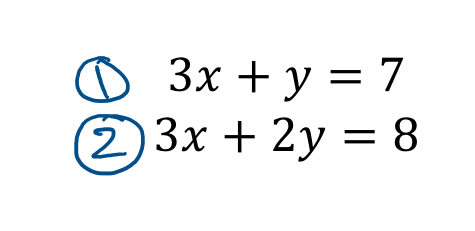CONTENT

• Solving Simultaneous Equations Using Elimination and Substitution Method
• Solving Equations Involving Fractions.
• Word problems.

#### SIMULTANEOUS LINEAR EQUATIONS

##### Methods of solving Simultaneous equation
1. Elimination method
2. Substitution method
3. Graphical method

Elimination method

One of the unknowns with the same coefficient in the two equations is eliminated by subtracting or adding the two equations. Then the answer of the first unknown is substituted into either of the equations to get the second unknown.

Example

Solve for x and y in the equations 2x + 5y   = 1 and 3x – 2y   =   30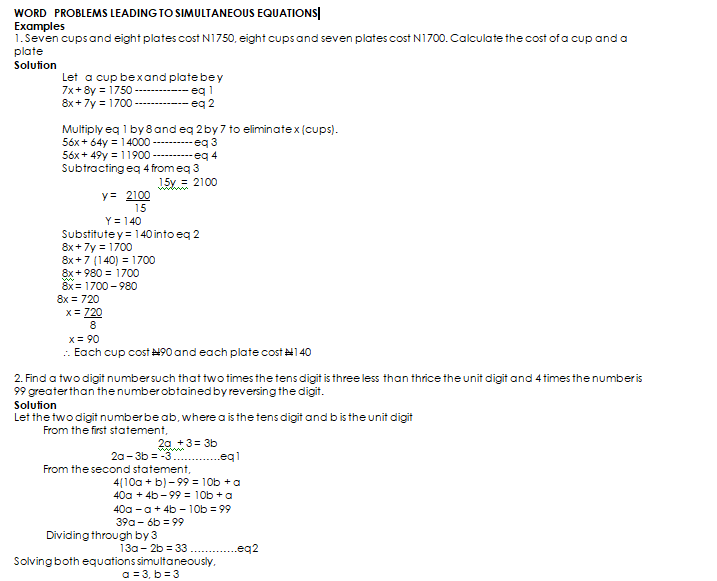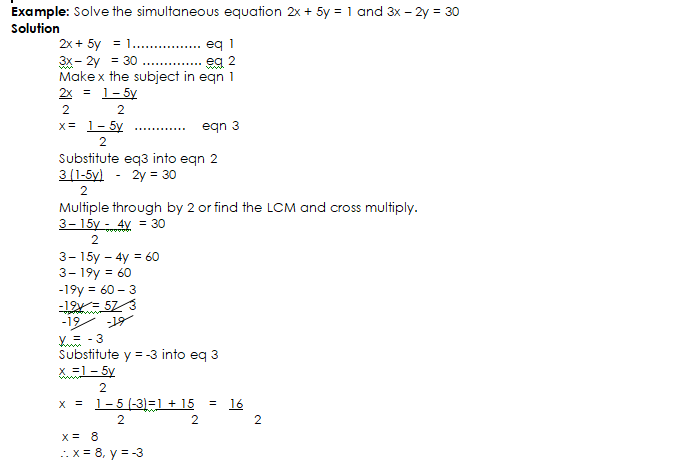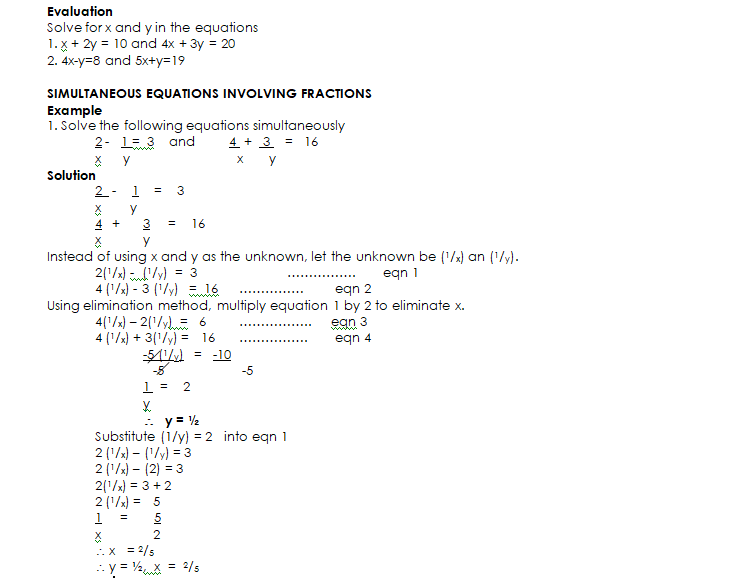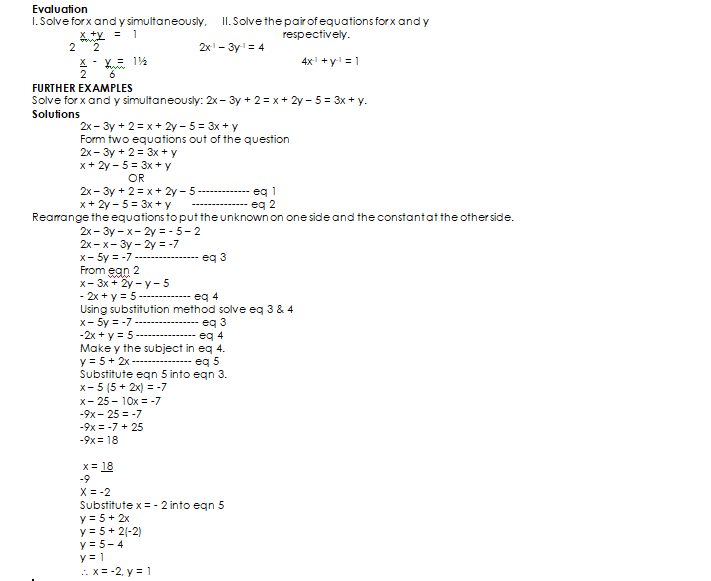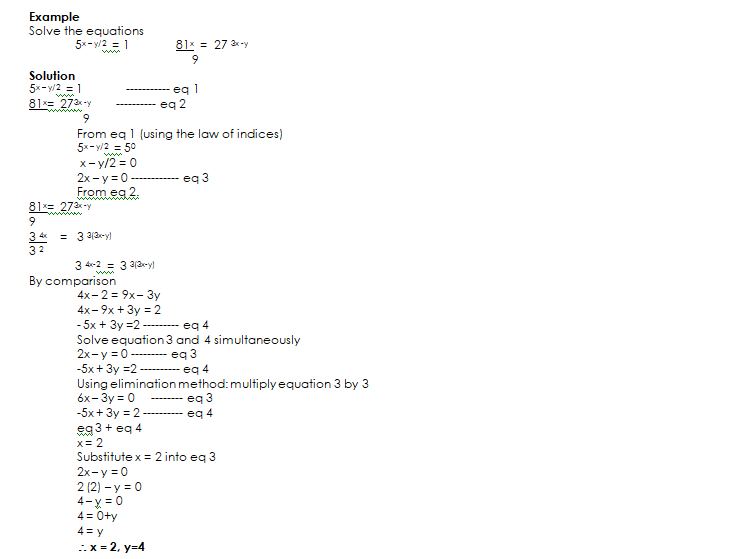###### GENERAL EVALUATION
1. Solve the following simultaneous equation: 3(2x – y) = x + y + 5 &  5(3x  –  2y) = 2 (x –y) + 1
2. Five years ago, a father was 3 times as old as his son. Now, their combined ages amount to 110years. How old are they?
3. Solve simultaneously,   2x + 2y = 1; 32x+y = 27
4. Solve: 2x – 2y + 5 = 3x – 4y + 2 = -1

In our next class, we will be talking more about Simultaneous Equations.  We hope you enjoyed the class.

Should you have any further question, feel free to ask in the comment section below and trust us to respond as soon as possible.

How Can We Make ClassNotesNG Better - CLICK to Tell Us💃

1.